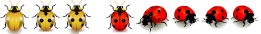KOA1
 Name:    KOA1

Multiple Choice
Identify the choice that best completes the statement or answers the question.

1.

Tell which math sentence is the same as the picture.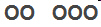a. 5 + 4 c. 2 + 3 b. 3 + 3 d. 1 + 3

2.

Tell which math sentence is the same as the picture.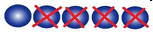a. 5 - 4 c. 4 + 1 b. 4 - 3 d. 1 + 5

3.

Tell which math sentence is the same as the picture.  OOOO  OOO
 a. 5 - 4 c. 4 + 3 b. 4 + 1 d. 3 + 3

4.

Tell which math sentence is the same as the picture.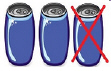a. 3 + 2 c. 1 - 0 b. 5 - 2 d. 3 - 1

5.

Tell which math sentence is the same as the picture.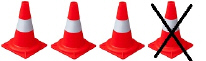a. 4 - 1 c. 2 + 2 b. 3 + 1 d. 8 + 4

6.

Tell which math sentence is the same as the picture.  \$\$\$\$    \$
 a. 4 - 1 c. 4 + 1 b. 5 - 1 d. 4 + 2

7.

Tell which picture shows the math sentence: 1 + 2
 a. X   XX c. XXX   XXXXX b. X   x d. X   XXX

8.

Tell which picture shows the math sentence: 2 + 2
 a.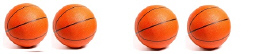c.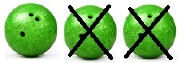b.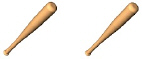d.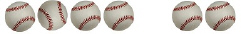Multiple Response
Identify one or more choices that best complete the statement or answer the question.

9.

Tell which picture goes with the math sentence: 5 + 4?
 a.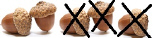c.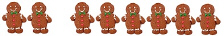b.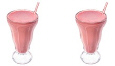d.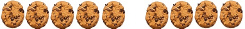10.

Tell which picture goes with the math sentence: 7 - 3?
 a.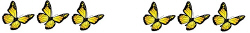c.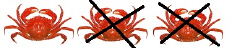b.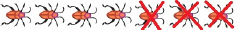d.